# Electronic DC Voltmeter

The electronic voltmeters are most widely used now a day, with the advancement of semiconductor devices they are becoming increasingly popular. Now, why do we use electronic voltmeters instead we have cheap electromechanical voltmeter available?? The answer is that they posses certain advantages so that they are popular. There are many types of electronic voltmeters available and accordingly we choose what we want. These types of voltmeters are constructed by the use of PMMC meters, rectifiers, voltage dividers, amplifiers, so that the current is directly proportional to the deflecting torque.

## Description of the Components used in DC Voltmeter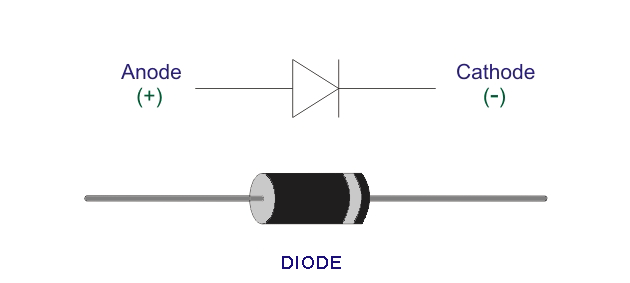### Diode

The diode is a two terminal device which is capable of blocking the current when it is in reverse biased condition. It conducts when it is in forward biased state. So this arrangement is used when we want to find out the average of the AC voltage.

The AC voltage is first rectified and then there we have to connect a PMMC instrument in order to measure the average voltage.

### Transistor

The transistor is a three terminal device which is used for amplification purpose. When a signal is applied to its base, then the signal is amplified by it as it has low input resistance and high output resistance when connected to active region.

It can also be operated as a switch. It has three modes of operation, namely-

1. Active Region.
2. Saturation Region.
3. Cut Off Region.

In the active region it has its input as forward biased and the output is reversed biased.
In the saturation region, both input and output are in forward biased mode.
In the cut-off region, input and output are in reversed biased state.
However the transistor has a considerable loss of power for that we use the transistor which has high input impedance. The types of transistors use when the loss has to be low are-
Field effect transistor or FET and Metal oxide semiconductor field effect transistor or MOSFET.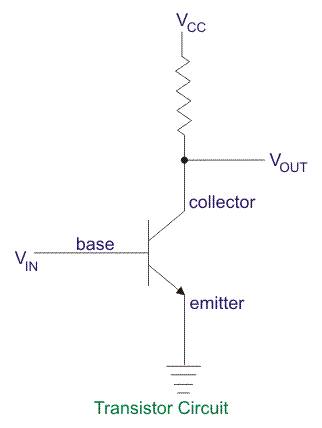### Amplifier

For a small signal we have to use amplifier in order to measure it. The amplifier generates the power required for measuring instruments so that there will not be any loading effect in the circuit and the original signal is retained after measurement also. Without the use of amplifiers we cannot measure very small signals typically used in communication circuits where the voltage level and power levels are very small.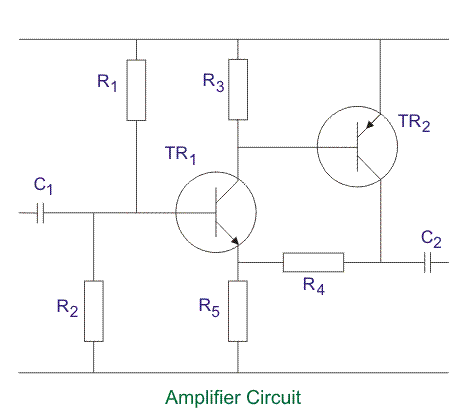### Voltage Divider

The voltage divider is provided in order to have the voltage tapping. Suppose the voltage we want is less then we go for voltage divider by connecting series resistance and output is taken across the desired resistor. Also, if we want to adjust the offset voltage, then also we can provide a potentiometer type arrangement in order to nullify the offset.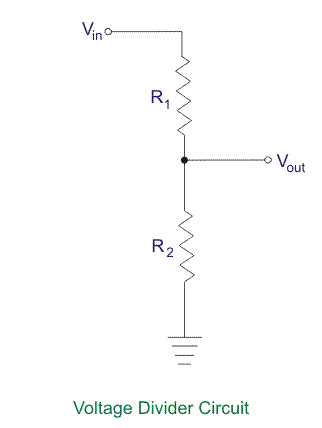### PMMC Meter

The instrument is used to indicate movement of the instrument that is to measure the current in the circuit. In this type of circuit, we use a taut suspension but in case of the other measurement types use pivot and jewel bearing arrangements. This is called the analog mode of operation, however if we want digital data we have to use A/D converter in order to have the digital reading. The main advantage of taut and suspension arrangement is that we do not require jewel bearings and the moving element is balanced. Jewel bearing has certain disadvantages such as friction is more due to damaged pivots or distorted control springs.

## Average Reading Diode Vacuum Tube Voltmeter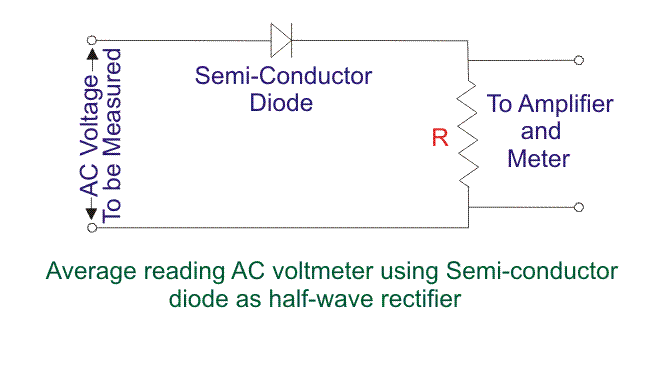The circuit is used to measure the average of AC voltage. Value of R should be very high in order to have almost all the voltage drop across the resistor so that it can be fed to amplifier and meter.

1. Simple Construction.
2. High resistance at the input so the power consumption is lower.

1. Low bandwidth.
2. Non linear operation when the voltage is low.

## Difference Amplifier type Electronic Voltmeter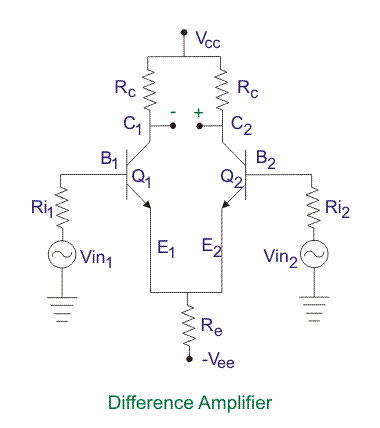The main principle of difference amplifier is that it amplifies the difference of the signal applied at both ends. Here, transistor is used but for better results we may use FET as well. In the output terminal we can connect a PMMC meter in order to get the reading. The transistor should be perfectly matched so that we get zero offset, if they are not matched then an offset null can be done by the use of a voltage divider across Vcc. When a PMMC meter is connected at the output and the voltage being applied at one end and base of transistor 2 is grounded say, then meter will deflect in proportion to the voltage applied at the base of first transistor so we get the reading in the meter. The resistance may be connected in series with the PMMC meter in order to limit the current.

Low Level Signal Detection
If the signal levels were low then the other mechanical deflection types of instruments produce a loading effect that means the instrument will consume an appreciable amount of power and there is a high chance that the signal may be lost, but electronic instruments are capable of providing power so that we can measure a voltage in terms of micro volts also.
Low Power Consumption
The power consumed by these instruments are low is as they can provide very high input impedance up to a few hundred mega ohms so the current in the circuit is much small and hence the power loss is also reduced.
High Frequency Range
In the electronic voltmeter, the measurement is almost independent of the frequency of operation. They have a very high range of operating frequencies up to a few Mega Hertz. The frequency response is much wider as the capacitance of the input circuit is very small.

High Cost
The cost of electronic voltmeters is very high as there are a lot of components involved such as a diode, transistors, resistors etc. On the other hand, PMMC instruments have low cost.
Damage
As we know, in case of electronic circuits, if one component is damaged by some means then the whole circuit should be replaced, this disadvantage also holds good in electronic voltmeter.

Want To Learn Faster? 🎓
Get electrical articles delivered to your inbox every week.
No credit card required—it’s 100% free.